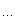# Set Up a View Matrix

(^_^,只能看到projection以后棱锥上下截面间的部分)
The three input vectors represent the following, respectively:

1. The eye point: [0, 3, -5].     (眼睛)
2. The camera look-at target: the origin [0, 0, 0]. (眼睛要看的东东)
3. The current world's up-direction: usually [0, 1, 0].   (指出那边是上面)using  Microsoft.DirectX.Direct3D;Device device
=   null ;   //  Create rendering device.//  Set up the view matrix. A view matrix can be defined given an eye point,//    a point to view, and a direction for which way is up. Here, you set//    the eye five units back along the z-axis and up three units, view the//    origin, and define "up" to be in the y-direction.device.Transform.View
=  Microsoft.DirectX.Matrix.LookAtLH(new  Vector3( 0.0f 3.0f - 5.0f ),new  Vector3( 0.0f 0.0f 0.0f ),new  Vector3( 0.0f 1.0f 0.0f ));# Set Up a Projection Matrix

(project以后得到我们能够看到的所有:一个棱锥上下截面间的部分)

This example demonstrates how to set up the projection transformation matrix, which transforms 3-D camera or view space coordinates into 2-D screen coordinates.

See the following C# code example, the Projection transformation matrix is set to be equal to the left-handed (LH) PerspectiveFovLH matrix. Input arguments to PerspectiveFovLH are as follows.

1. Field of view in radians: pi/4. (一般都为1/4 pi)
2. Aspect ratio, or view-space height divided by width: 1, for a square window. (长宽的比)
3. Near clipping plane distance: 1 unit.     (离眼睛近的点,即棱锥的上面)
4. Far clipping plane distance: 100 units.  (离眼睛远的点,即棱锥的下面)using Microsoft.DirectX;Direct3D.Device device
= null;  // Create rendering device.// For the projection matrix, you set up a perspective transform (which//   transforms geometry from 3-D view space to 2-D viewport space, with//   a perspective divide making objects smaller in the distance). To build//   a perspective transform, you need the field of view (1/4 pi is common),//   the aspect ratio, and the near and far clipping planes (which define//   the distances at which geometry should no longer be rendered).device.Transform.Projection
= Matrix.PerspectiveFovLH((
float)Math.PI / 41.0f1.0f100.0f );## What Is a World Transformation?

A world transformation changes coordinates from model space, where vertices are defined relative to a model's local origin, to world space, where vertices are defined relative to an origin common to all of the objects in a scene. In essence, the world transformation places a model into the world; hence its name.

## Setting Up a World Matrix

As with any other transformation, you create the world transformation by concatenating a series of transformation matrices into a single matrix that contains the sum total of their effects. In the simplest case, when a model is at the world origin and its local coordinate axes are oriented the same as world space, the world matrix is the identity matrix. More commonly, the world matrix is a combination of a translation into world space and possibly one or more rotations to turn the model as needed.

The following C# code example, from a fictitious 3-D model class written in C#, creates a world matrix that includes three rotations to orient a model and a translation to relocate it relative to its position in world space.public class ModelClass{private float xPos=0;private float yPos=0;private float zPos=0;private float Pitch=0;private float Yaw=0;private float Roll=0;//Other model properties and methodspublic Matrix MakeWorldMatrix(Matrix worldMatrix){worldMatrix.Translate(xPos,yPos,zPos);Matrix matRot
= Matrix.Identity;matRot.RotateYawPitchRoll(Yaw,Pitch,Roll);worldMatrix
= Matrix.Multiply(matRot, worldMatrix);return worldMatrix;}}

Note: Direct3D uses the world and view matrices that you set to configure several internal data structures. Each time you set a new world or view matrix, the system recalculates the associated internal structures. Setting these matrices frequently—for example, thousands of times per frame—is computationally time-consuming. You can minimize the number of required calculations by concatenating your world and view matrices into a world-view matrix that you set as the world matrix, and then setting the view matrix to the identity. Keep cached copies of individual world and view matrices so that you can modify, concatenate, and reset the world matrix as needed. For clarity, Direct3D samples in this documentation rarely employ this optimization.

posted on 2006-06-09 15:07 梦在天涯 阅读(1447) 评论(0)  编辑 收藏 引用 所属分类: DirectX### 公告

EMail：itech001#126.com

• 随笔 - 461
• 文章 - 4
• 评论 - 746
• 引用 - 0

•

• 积分 - 1747832
• 排名 - 5